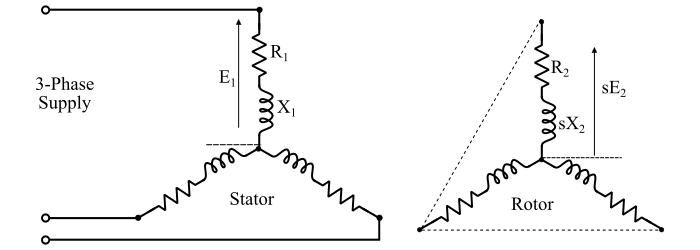# 3-Phase Induction Motor Rotor Frequency, EMF, Current and Power Factor

## Rotor Current Frequency

The frequency of current and voltage in the stator of a 3-phase induction motor must be same as the supply frequency and is given by,

$$\mathrm{𝑓 =\frac{𝑁_{𝑆}𝑃}{120}… (1)}$$

But, the frequency of the current and EMF in the rotor circuit of the 3-phase induction motor is variable and depends upon the difference between the synchronous speed (NS) and the rotor speed (Nr), i.e., on the slip. Thus, the rotor frequency is given by,

$$\mathrm{𝑓_{𝑟} =\frac{(𝑁_{𝑆} − 𝑁_{𝑟} )𝑃}{120}… (2)}$$

Now, from the equations (1) and (2), we get,

$$\mathrm{\frac{𝑓_{𝑟}}{𝑓}=\frac{𝑁_{𝑆} − 𝑁_{𝑟}}{𝑁_{𝑆}}}$$

$$\mathrm{∵ \:Slip,\:𝑠 =\frac{𝑁_{𝑆} − 𝑁_{𝑟}}{𝑁_{𝑆}}}$$

$$\mathrm{∴ 𝑓_{𝑟} = 𝑠𝑓 … (3)}$$

i. e. , Rotor Current Frequency = Per unit slip × Supply frequency When the rotor is stationary, i.e., Nr = 0, then,

$$\mathrm{𝑠 =\frac{𝑁_{𝑆} − 𝑁_{𝑟}}{𝑁_{𝑆}}= 1}$$

$$\mathrm{∴ \: 𝑓_{𝑟} = 𝑓}$$

Hence, when the rotor is stationary, the frequency (fr) of the rotor current is the same as that of the supply frequency (f).

When the rotor picks up speed, the relative speed between the rotating magnetic field and the rotor decreases. As a result of this, the slip (s) and hence the rotor current frequency decreases.

At synchronous speed, i.e., Nr = NS,

$$\mathrm{𝑠 =\frac{𝑁_{𝑆} − 𝑁_{𝑟}}{𝑁_{𝑆}}= 0}$$

$$\mathrm{∴ 𝑓_{𝑟} = 0}$$

## Rotor EMF

When the rotor is stationary, the 3-phase induction motor behaves as a 3-phase transformer with secondary winding short circuited. Thus, the per phase induced EMF in the rotor (or secondary) is given by,

$$\mathrm{Rotor\:EMF/Phase,\:𝐸_{2} = 𝐸_{1} × \frac{𝑁_{2}}{𝑁_{1}}= 𝐾\:𝐸_{1} … (3)}$$

Where,

• E1 = Per phase stator voltage.
• N1 = Number of turns in stator winding per phase.
• N2 = Number of turns in rotor winding per phase.

When the rotor is running at slip ‘s’, then the relative speed between the rotating magnetic field of the stator and the rotor is (NS – Nr). Therefore, the rotor EMF is directly proportional to the (NS – Nr) or slip (s), i.e.

$$\mathrm{Rotor\:EMF/Phase\:at\:slip\:𝑠,\:{𝐸^{′}_{2}} = 𝑠\:𝐾\:𝐸_{1} … (4)}$$

## Rotor Current and Power Factor

Consider a 3-phase induction motor at any slip value ‘s’ as shown in the figure below.Here, the rotor is assumed to be wound rotor and is connected in star. Therefore,

$$\mathrm{Rotor\:EMF\:Phase,𝐸^{′}_{2} = 𝑠\:𝐸_{2 }\:\:\:(let\:𝐾 = 1)}$$

$$\mathrm{Rotor\:reactance/Phase,{𝑋^{′}_{2}} = 𝑠\:𝑋_{2}}$$

Where, X2 is the rotor reactance per phase at standstill condition.

The resistance of the rotor circuit is R2 per phase and is independent of the frequency and hence does not depend upon slip. Similarly, the resistance (R1) and reactance (X1) of the stator winding do not depend upon slip.

Since the 3-phase induction represents a balanced 3-phase load, then we need to analyse one phase only and the conditions in the other two phases being similar.

### Case 1 – When the rotor is stationary

When the rotor is stationary, the motor is at standstill (slip, s = 1)$$\mathrm{Rotor\:current/phase ,\:𝐼_{2} =\frac{𝐸_{2}}{𝑍_{2}}=\frac{𝐸_{2}}{\sqrt{{𝑅_{2}}^{2} + {𝑋_{2}}^{2}}}… (5)}$$

$$\mathrm{Rotor\:Power\:Factor,\:cos \:φ_{2}=\frac{R_{2}}{𝑍_{2}}=\frac{R_{2}}{\sqrt{{𝑅_{2}}^{2} + {𝑋_{2}}^{2}}}… (6)}$$

### Case 2 – When the motor is running at slip ‘s’$$\mathrm{Rotor\:current/phase,\:{𝐼^{′}_{2}}=\frac{𝑠\:𝐸_{2}}{𝑍^{′}_{2}}=\frac{𝑠\:𝐸_{2}}{\sqrt{{R_{2}}^{2} +(SX_{2})^{2}}}}…… (7)$$

$$\mathrm{Rotor\:Power\:Factor,\:cos\:{φ^{′}_{2}} =\frac{R_{2}}{𝑍^{′}_{2}}=\frac{R_{2}}{\sqrt{{𝑅_{2} }^{2}+(SX_{2})^{2}}}}… (8)$$

## Numerical Example 1

A 3-phase, 50 Hz induction motor has 8 poles and operates with a slip of 4 % at a certain load. Determine the frequency of the rotor current.

## Solution

$$\mathrm{Rotor\:current\:frequency, 𝑓_{𝑟} = 𝑠\:𝑓 = 0.04 × 50 = 2\:Hz}$$

## Numerical Example 2

A 3-phase, 4-pole induction motor is connected to a 50 Hz supply. The voltage induced in the rotor bar conductors is 5 V when the rotor is at standstill. Calculate the voltage and frequency induced in the rotor conductors at 500 RPM.

## Solution

$$\mathrm{Synchronous\:speed,\:𝑁_{𝑆} =\frac{120\:𝑓}{𝑃}=\frac{120 × 50}{4}= 1500\:RPM}$$

$$\mathrm{Slip,\:𝑠 =\frac{𝑁_{𝑆} − 𝑁_{𝑟}}{𝑁_{𝑆}}=\frac{1500 − 500}{1500}= 0.67}$$

Therefore, corresponding to the slip ‘s’,

$$\mathrm{Induced\:voltage\:in\:rotor,\:𝑉^{′}_{2} = 𝑠\:𝑉_{2} = 0.67 × 5 = 3.35\:V}$$

$$\mathrm{Rotor\:frequency,\:𝑓_{𝑟} = 𝑠\:𝑓 = 0.67 × 50 = 33.5\:Hz}$$

## Numerical Example 3

An 8-pole, 3-phase, 50 Hz induction motor is running at full load with a slip of 5 %. The rotor is star connected and its per phase resistance and standstill reactance are 0.35 Ω and 2 Ω respectively. The EMF between slip rings is 150 V. Determine the rotor current per phase and rotor power factor. Assuming the slip rings are short circuited.

## Solution

The per phase rotor EMF at standstill is,

$$\mathrm{𝐸_{2} =\frac{150}{\sqrt{3}}= 86.61\:V}$$

$$\mathrm{Rotor\:EMF/phase\:at\:full\:load,\:𝐸^{′}_{2} = 𝑠\:𝐸_{2} = 0.05 × 86.61 = 4.33\:V}$$

$$\mathrm{Rotor\:reactance/phase\:at\:full\:load,\: 𝑋^{′}_{2} = 𝑠\:𝑋_{2} = 0.05 × 2 = 0.1\:Ω}$$

$$\mathrm{Rotor\:impedance/phase\:at\:full\:load, \:𝑍^{′}_{2}=\sqrt{{0.35}^{2} + {0.1}^{2}}= 0.364\:Ω}$$

Therefore,

$$\mathrm{Rotor\:current/phase =\frac{𝐸^{′}_{2}}{𝑍^{′}_{2}}=\frac{4.33}{0.364}= 11.895\:A}$$

$$\mathrm{Rotor\:Power\:Factor =\frac{𝑅_{2}}{𝑍^{′}_{2}}=\frac{0.35}{0.364}= 0.96\:(lagging)}$$